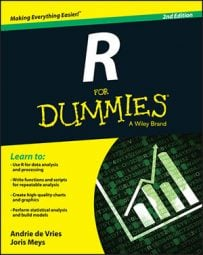##### R For DummiesVectors have a structure and a type, and R is a bit sensitive about both. Feeding R the wrong type of vector is like trying to make your cat eat dog food: Something will happen, and chances are that it won’t be what you hoped for. So, you’d better know what type of vector you have.

## Looking at the structure of a vector

R gives you an easy way to look at the structure of any object. This method comes in handy whenever you doubt the form of the result of a function or a script you wrote. To take a peek inside R objects, use the str() function.

The str() function gives you the type and structure of the object.

Take a look at the vector baskets.of.Granny:

```> str(baskets.of.Granny)
num [1:6] 12 4 5 6 9 3```

R tells you a few things here:

• First, it tells you that this is a num (numeric) type of vector.

• Next to the vector type, R gives you the dimensions of the vector. This example has only one dimension, and that dimension has indices ranging from 1 to 6.

• Finally, R gives you the first few values of the vector. In this example, the vector has only six values, so you see all of them.

If you want to know only how long a vector is, you can simply use the length() function, as follows:

```> length(baskets.of.Granny)
 6```

Vectors in R can have other types as well. If you look at the vector authors, for example, you see a small difference:

```> authors <- c("Andrie", "Joris")
> str(authors)
chr [1:2] "Andrie" "Joris"```

Again, you get the dimensions, the range of the indices, and the values. But this time, R tells you the type of vector is chr (character).

Here are some vectors you will want to know:

• Numeric vectors, containing all kinds of numbers.

• Integer vectors, containing integer values. (An integer vector is a special kind of numeric vector.)

• Logical vectors, containing logical values (TRUE and/or FALSE).

• Character vectors, containing text.

• Datetime vectors, containing dates and times in different formats.

• Factors, a special type of vector to work with categories.

All of the listed types of vectors may have missing values (NA).

R makes clear distinctions among these types of vectors, partly for reasons of logic. Multiplying two words, for example, doesn’t make sense.

## Testing vector types

Apart from the str() function, R contains a set of functions that allow you to test for the type of a vector. All these functions have the same syntax: is, a dot, and then the name of the type.

You can test whether a vector is of type foo by using the is.foo() function. This test works for every type of vector; just replace foo with the type you want to check.

To test whether baskets.of.Granny is a numeric vector, for example, use the following code:

```> is.numeric(baskets.of.Granny)
 TRUE```

You may think that baskets.of.Granny is a vector of integers, so check it, as follows:

```> is.integer(baskets.of.Granny)
 FALSE```

R disagrees with the math teacher here. Integer has a different meaning for R than it has for us. The result of is.integer() isn’t about the value but about the way the value is stored in memory.

R has two main modes for storing numbers. The standard mode is double. In this mode, every number uses 64 bits of memory. The number also is stored in three parts. One bit indicates the sign of the number, 52 bits represent the decimal part of the number, and the remaining bits represent the exponent. This way, you can store numbers as big as 1.8 × 10308 in only 64 bits.

The integer mode takes only 32 bits of memory, and the numbers are represented as binary integers in the memory. So, the largest integer is about 2.1 billion, or, more exactly, 231 – 1. That’s 31 bits to represent the number itself, 1 bit to represent the sign of the number, and –1 because you start at 0.

You should use integers if you want to do exact integer calculations on small integers or if you want to save memory. Otherwise, the mode double works just fine. One of the nice things about R is that you hardly ever need to worry about whether something is stored as an integer or a double!

You force R to store a number as an integer by adding L after it, as in the following example:

```> x <- c(4L, 6L)
> is.integer(x)
 TRUE```

Whatever mode is used to store the value, is.numeric() returns TRUE in both cases.